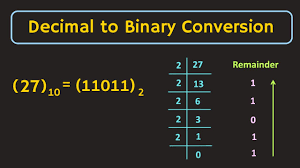September 23, 2023
Education

# Decimal to BinaryA number system can be expressed in various ways. Some of the systems are the binary number system, decimal number system, octal number system, and so on. The conversion of decimal to binary can be done through different methods. One of the easiest methods to convert a decimal to binary is by dividing the decimal number by 2. This can be done recursively until you get the appropriate answer.We will cover some topics such as, what are decimals, types of decimals, conversion of decimals into a fraction, Let’s learn how to convert decimal to binary.

• Step 1: Suppose we want to convert 1010 into binary.
• Step 2: Divide 10 by 2 to get quotient 5 and remainder 0, where 0 is the binary number.
• Step 3: Now divide quotient 5 and check with the remainder.
• Step 4: Repeat the steps until the quotient is equal to 0.
• Step 5: Take remainders from LSB TO MSB.

## Decimal

In mathematics, you can find various types of numbers, such as real numbers, whole numbers, fractions, irrational and rational numbers, and so on. Decimal can be defined as the set of numbers that lie between the integers on a number line. A line that consists of all real numbers except the complex number is known as the number line. The decimals are used to represent or express the fractions and whole numbers. We can say that the use of decimal is a different way of expressing fractions. A part of the whole that comprises a numerator (upper part) and a denominator (lower part) is known as the fraction. All the positive integers including the number zero are defined as whole numbers. Decimals use a point ‘.’ known as a decimal point which is used to segregate a whole number from a fraction. The numbers situated on the left-hand side of the decimal point are referred to as fraction or whole numbers and the numbers situated on the right-hand side of the point are denoted as decimal fractions. Some examples of decimals are as follows, 4.2, 3.6, 2.5, and many more. The most significant use of decimal is to give precise values on the quantities which can be measured such as length, weight, height, distance, money, etc.

## Types of Decimals

To recall, decimals are defined as the set of numbers that lie between the integers on a number line. There are various types of decimals distinguished based on the repetition of a digit. Some of them are, recurring decimal, non-recurring decimal, terminating decimal, etc.

• A type of decimal that does not occur after a set of a finite number of decimals can be defined as the terminating decimal. For example, 27.2, 0.2, 0.5, and so on.
• A type of decimal that does re-occur after a set of a finite number of decimals is defined as the non-terminating decimal. Some examples of the non-terminating decimals are as follows, 827.927…., 3.142…..
• Non-terminating decimals are further classified into two types. They are, recurring non-terminating decimal numbers and the non-recurring non-terminating decimal number.
• The type of non-terminating decimal number where the digits repeat after a fixed interval is known as the recurring decimal number. Likewise, when the digits do not repeat after a fixed interval, the decimal number is known as the non-recurring decimal number.

## Decimals into Fractions

A set of numbers that are represented as a part of the whole is known as the fractions. The steps of converting a decimal into a fraction are:.

• The first step is to determine the place value of the given digits after the decimals.
• Then, determine the value of the denominator using the help of the first step.
• Now, remove the point known as the decimal point and write the number as a fraction.
• You may simplify the fraction further if required.

You may follow the above methods in the correct order to convert a decimal into a fraction.

If you want to study the topics mentioned above in a detailed, fun, and interactive manner, then visit Cuemath to book a free session.